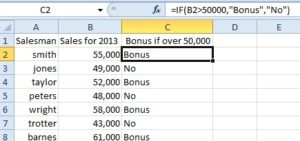### Give us a call

Our team of experts are on hand and ready to help.### Live chat

Ready to chat data? That's what we're here for### Submit an enquiry

Fill out your details and one of the team will be in touch

Using the IF function in Excel can be very useful in a spreadsheet. The IF function will allow you to check if a condition you have set is true or false. You can use the IF function in a formula to give a result, depending on whether the data expressed in a cell meets the criteria.

For example, you could use the IF function in a spreadsheet of sales results and set the condition that if a salesman reaches a certain target, they will get a bonus. The IF function formula for the sales spreadsheet will look like this,=IF(A1>50000,”Bonus”,”No”)

There are three parts of the IF function, the logical test, the value if true and the value if false, so the basic IF function will look like this, =IF(logical_test,value_if_true,value_if_false,)

The logical test is the argument that you want the test for, is the number in the cell A1, greater than 50,000. For our example we use the (>) Greater than conditional operator.The ”Bonus is if the value is true and “No” is if the value is false.

This is a basic IF function formula, but you can use other conditional operators in the formula.

< Less than

>= Greater than or equal to

<= Less than or equal to

<> Not equal to

In more complex IF function formulas, you are able to use a combination of these conditional operators.## Our blog

Get up to speed on what’s happening in the world of Data…